Volume Definition, Formulas & more - Homework Help

# Learn everything from the definition of Volume to it’s Unit & Formulas

## Top Questions

t schedule 3 people for the day my % scheduled is 78.57% My question is how did the computer come up with the 78.57%?
View More

I would love to get a written solution. I did the other proof tree that you can see in the attaached files. So its supposed to look like that Thank You.
View More

xtbook. i really need in in half an hour
View More

## Learn everything from definition of Volume to formulas:

### Volume Defination:

Volume  is defined as the space occupied by 3-Dimensional objects. For example, volume of the cylinder is defined as the space occupied by the 3-Dimensional shape of the cylinder.

## What are the formulas to calculate volume of common shapes around us:

### Volume of Cube

Cube is the 3-D shape of the square. Volume of the cube can be given by the formula as under :-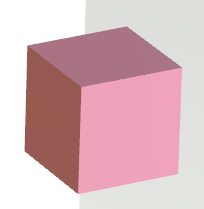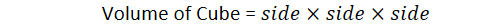### Volume of Cuboid

Cuboid is the 3-D shape of a rectangle. Volume of the cuboid is given by formula as under:-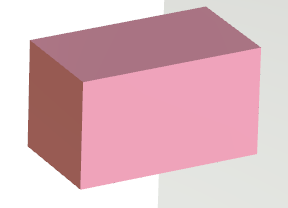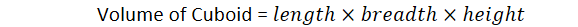### Volume of Sphere

Sphere is the 3-D shape of Circle . Volume of sphere can be given by formula as under:-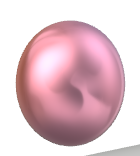### Volume of Cylinder

Volume of cylinder is given by the formula as under: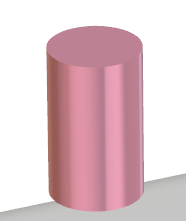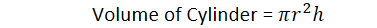### Volume of Right Circular Cone

Volume of Right Circular Cone can be given by formula as under :-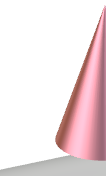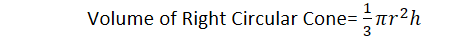## Volume Questions & Answers - Sample

Que 1 :- Calculate volume of a cylindrical bucket having radius 5 cm and height 2 cm.

Ans  Height of cylinder, h = 2 cm

Radius of cylinder, r = 5 cm

As,Volume of cylindrical bucket = 22(5) = 20 cm3

Que 2 :- What is the volume of the right circular cone of radius 8 m and height 6 m ?

Ans Height of the right circular cone, h = 6m

Radius of the right circular cone, r = 8 m

As,Thus , volume of the right circular cone = 13(8)2(6)

=  (8)2(2)

=  128 m^3

As,Volume of sphere =   4/3π(3)^3 = 4π×3×3

= 36πcm^3

## Need help in understanding the world of volume and everything around it?

In the United States and Canada, typically as per school curriculum the basic geometrical concepts such as Area, Perimeter and Volume are introduced in Grade 5. This is the first time students learn fun facts about the topic of volume such as – all sides of a cube are equal and the volume is a simple cube of the length of its side.

Other than learning these fun facts, volume doesn’t  just introduce students to the three dimensional shapes but it also brings the more complex topics for middle school students such as different shapes have different volumes and the fact that there are multiple ways of measuring volume. One can measure the volume by the shape but in other cases we can use the density of the material to also calculate the volume. Also the fact that state of matter also matter as solids are just packed but liquids end up taking the share of the 3 dimensional space.

The topic of volume can easily get tricky for students and giving them the correct support and experiences with tools can be helpful. Conceptual understanding of volume and different types of shapes can help motivate students to learn & focus on the volume formulas.

Our Homework Help is designed to help middle & high school students to understand the details about the topic of volume. Our team of Math tutors is committed towards providing our students the details they need to develop an understanding of the core concepts of volume.

Available 24/7, our tutors can handle all volume related questions and get you the correct solutions at the most reasonable prices.

## Follow these steps to available homework help at TutorEye:

Step 1: Fill out the help homework form & post your homework questions

Step 2: Choose from the multiple requests that fit your budget and timeline.

Our work is 100% original and we stand behind the quality of support and guidance provided. Our tutors help hundreds of kids on a daily basis to understand difficult concepts, find solutions and improve Math grades.

Question 1: What is the formula for volume?

The volume of the three dimensional figure is the space enclosed inside it, the volume is measured in cubic units. The basic principle to calculate the volume of the cuboid is length × width × height or we can say the area of the base multiplied by the height. We can use the same concept to calculate the area of the other three dimensional figure. Some of the volumes of the three dimensional figures are given as

 SHAPE TOTAL SURFACE AREA Cube a^3 Sphere 4/3 π ×(radius)^3 Cylinder π ×r^2 ×h Cuboid l ×b ×h Cone 1/3 ×π ×(r)^2h

Question 2: How do you find volume in a math problem?

To find the volume of a given figure we need to first find out the dimensions of the given figure and use the formula of volume for that given figure. For example, to calculate the volume of the cube we need to know the side of the cube and then we use the formula of volume for the cube which is equal to a3, similarly for the cuboid if we know the length, breadth and height we can calculate the volume by using the formula length × breadth × height. So the basic principle we can use to calculate volume is the area of the base times the height.

Question 3: How do you explain volume?

Volume is the amount of space contained in a three dimensional figure. Take an example of a cylindrical jar that has two parallel circular bases joined by a curved surface, if you fill up the jar with water the amount of water completely filled in that jar represents the volume of the cylindrical jar. Similarly, if you take a spherical ball and fill it with the air completely then the air occupied by the spherical ball is the total volume of the spherical ball.

Question 4: Does volume mean add?

Volume of the three dimensional figure is the quantity contained in it, we can calculate the volume of any three dimensional figure by first calculating the area of the base and then adding them all, we can add the area of base as many times as the height is, taking an example of cuboid the area of the base is l × b and adding this base area height times will give the volume which is l × b × h.  In this way we can say that the volume of the three dimensional figure is also calculated using adding.

Question 5: How to find volume?

In order to find the volume of the three dimensional figure we need to find the dimensions of the three dimensional figure like length multiplying the length with the width and the result being multiplied by the height gives the volume of the three dimensional figure.

Similarly we can find out the volume of other figures if we know the dimensions of the given figure. We can also multiply the area of the base with height which can help us in calculating the area of any three dimensional figure.

Question 6: What is volume?

Volume is the total space contained in the three dimensional figure, we can also define the volume as the amount of any matter that can be filled in that object. Another word that is similar to volume is capacity. It is measured in cubic units. Different shapes have different formulas to calculate the volume of the figure. We need the dimensions in order to use formulas to calculate the volume.

Question 7: How to find the volume of a cylinder?

A circular cylinder is the three dimensional figure having two base circles which are parallel to each other and are joined by the curved surface area. The volume of the figure can be calculated using the basic principle by first calculating the area of the base and multiplying it by the height. The area of the base circle is pi × (radius)2  and height is h

Hence the volume is the area  of base ×height   = π ×(radius)^2 ×height.

Question 8: How to calculate the volume?

In order to calculate the volume of the three dimensional figures we can use the formula of volume for different figures. We need to know the dimensions of the three dimensional figure to calculate the volume. Or if you have the area of base of the given three dimensional figure when it is multiplied by the height it gives the volume of the figure. The units of volume are in cubic units.

Question 9: How to find the volume of a rectangular prism?

A rectangular prism consists of six rectangular faces that are perpendicular to each other. We can calculate the volume of the rectangular prism by first calculating the area of the base and then multiplying with the height. The area of the base will be equal to the length × breadth, then the volume of the rectangular prism will be  (area of base) × height
= length × breadth × height = l ×b ×h  where (l is the length, b is the breadth, h is height)

We can observe that the volume of the cone is one- third of the volume of the cylinder.

Question 10: How to find the volume of a cone?

A cone is the three dimensional figure whose base is the circle and it tapers from base to a point which is known as apex or vertex. We can calculate the volume of the cone using the basic principle that is multiplying the base area with height. For a given cone having radius r and height h then volume of the cone will be:

(1/3) × area of the base x height = (1/3) × π × r^2 ×h

Question 11: How to find the volume of a cube?

A cube is a three dimensional figure having six square faces having all sides equal to each other. The volume of a cube is the total space occupied by it, the formula to calculate the volume of the cube is multiplying the side three times. We can use the basic principle to calculate the volume of the cube, first calculate the area of the base of the cube which is equal to (side)2 then multiplying this by the height (which is equals to a ) = (side)2 ×side = (side)3

Question 12: How to find the volume of a pyramid?

A pyramid is a polyhedron having faces as polygons such as triangular pyramid, square pyramid, rectangular pyramid, pentagonal pyramid etc. in case of triangular pyramid the base is the triangle similarly in case of square pyramid the base is the square. The volume of the pyramid is the entire region between the faces. In order to find out the volume of the pyramid of base B and height h, we can first take a prism of base B and height h, the volume of the prism is B ×h and we know that the volume of the pyramid is one- third of the prism, hence the volume of pyramid = (1/3)×B×h

Question 13: What is volume in math?

In math volume is the total space enclosed by the boundary of the three dimensional figure. Let us take an example of cuboidal aquarium filled with water, the total amount of water contained in it gives the volume of this cuboidal aquarium, similarly a cylinder completely filled with gas will have a volume of total amount of air present in it. So we can say that the volume of any three dimensional figure is the total space present in it.

Question 14: What is volume measured in?

Volume is measured in cubic units, we can take an example of cuboid whose volume is to be find out, the formula for the volume is length(m) ×breadth(m) ×height(m), we know the SI unit of length, breadth and height is in meters, when we multiply them together we get cubic meters. Hence here the unit of volume is cubic meters if we take the length, breadth and height in centimeters we get the volume in cubic centimeters so we generalize the unit of volume as cubic units.

Question 15: What is the volume of a sphere?

The sphere is a three dimensional round figure; there are three axes present in it x, y and z. The relation between the volume of sphere, volume of cone, and volume of cylinder is:

Volume of Cylinder = Volume of Cone + Volume of Sphere ⇒ Volume of Sphere = Volume of Cylinder - Volume of Cone

As we know, the volume of cylinder = πr2h and volume of cone = one-third of the volume of cylinder = (1/3)πr^2h

The volume of Sphere = Volume of Cylinder - Volume of Cone ⇒ Volume of Sphere = πr^2h - (1/3)πr^2h = (2/3)πr^2h

In this case, height of cylinder = diameter of sphere = 2r

Hence, volume of sphere is (2/3)πr^2h = (2/3)πr^2(2r) = (4/3)πr^3